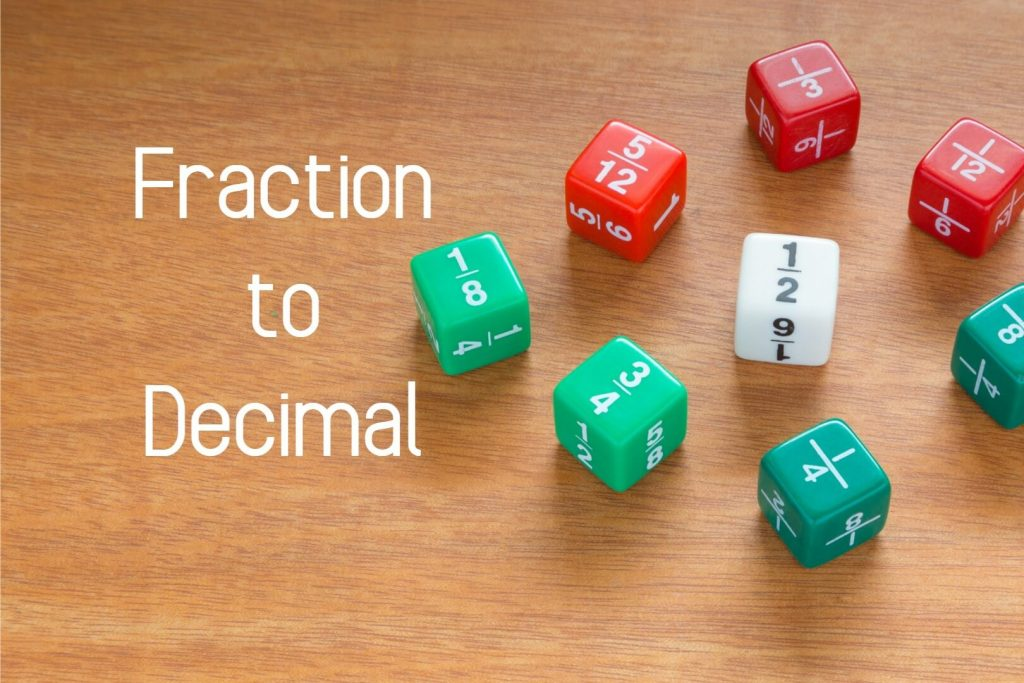# Fraction to Decimal

Decimals and Fractions are like sisters of the same family. If we know the decimal, we can find the fraction, and also when we know the fraction, we can find the corresponding decimal as well.

• How to convert Fraction to Decimal
• How to convert Decimal to Fraction

## Convert Fraction to Decimal

### (a) Fractions with 10 as the denominator

The shaded region can be express as a fraction

Then,

Like that,

If the denominator is 10 there will be only one decimal place after the decimal point.
The value in the first decimal place will be the numerator of the fraction.

### (b) Fractions with 100 as the denominator

The shaded part can be expressed as a fraction and it is,

Examples:

If the denominator is 100 there will be two decimal places after the decimal point.
The decimal value will be the numerator of the fraction.

### (c) Fractions with 1000 as the denominator

If the denominator is 1000 there will be three decimal places after the decimal point.
The decimal value will be equal to the numerator.

Examples:

### (d) Fractions having denominators that are not multiples of 10

Under this topic, we discuss examples whose denominators are not multiples of 10, but the ones we can convert into multiples of 10 easily by using Equivalent Fractions knowledge.

Example 01:

We can convert 5 into 10, 100, 1000, or any multiple of 10. The easiest one is converting it into 10.

Example 02:

4 cannot be converted into 10. But can be converted into any higher multiple of 10.
So, let’s convert 4 into 100.

Example 03:

Under converting fractions to decimals we can also discuss converting mixed numbers to decimals.

Let’s see some examples.

Example 01:

In this example what you have to do is, separate the whole number part and the fractional part and convert the fraction into a decimal.

When doing decimal addition you have to write place value correctly.

Example 02:

Example 03:

Example 04:

If you get any improper fraction, first convert it into a mixed number then convert it into a decimal.

You can find lessons on mixed numbers on our website from the following link.

Let’s see an example.

Sometimes you will get fractions having denominators that are unable to convert into multiples of 10.

For example,

If you get a fraction like this, you have to use the knowledge about division to convert this to decimal.

Let’s see.

We have to divide numerator by denominator by using long division.

As you can see,

You can round off this to the nearest second decimal place and you can write it as 0.67

## Convert Decimal to Fraction

This is easier than converting fractions to decimals.

You have to follow only a few simple rules.

• If you have one decimal place, denominator of the fraction will 10.
• If you have two decimal places, denominator of the fraction will be 100.
• Like wise if we have three decimal places, denominator will be 1000.

Let’s try to understand the idea through some examples.

Example 01:

0.6
Here we have only one decimal place. So the denominator will be 10.

Then what will be the numerator?

The numerator is the numerical value that we get after removing the decimal point.

Here in 0.6 -> 06 = 6

Therefore,

AS we know fractions have to be in their simplest form
So,

You can learn about HCF from the following article.

Example 02:

Example 03:

Example 04:

Example 05:

## Fraction to Decimal Calculator

Fraction to Decimal Calculator is an online tool that gives a person the ability to learn and understand more about the fraction they are dealing with. They are also a great tool for students who wish to improve their math skills. Learning and understanding decimals will greatly help students in their future education endeavors as well.

## Decimal to Fraction Calculator

Decimal to Fraction Calculators are an essential part of the field of mathematics and have applications in all areas of science. DFC is a tool that is extremely useful for students and teachers of mathematics, from primary school children to professional mathematicians and scientists.